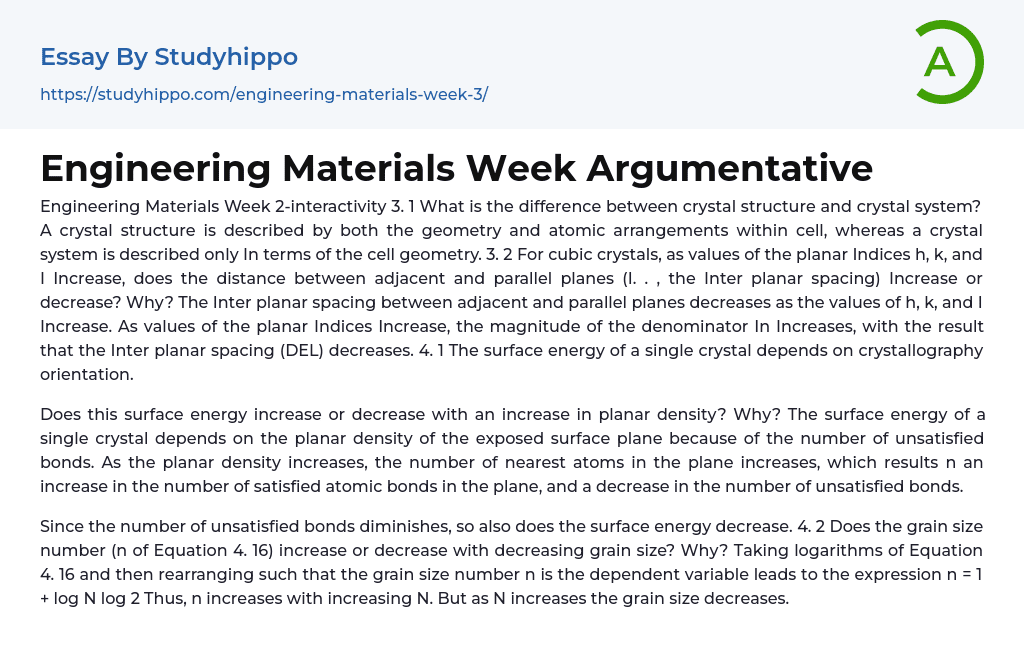# Engineering Materials Week Argumentative Essay Example

Available Only on StudyHippo
Topics:
• Pages: 1 (272 words)
• Published: December 21, 2017
• Type: Tests
Text preview

Engineering Materials Week 2-interactivity

What is the difference between crystal structure and crystal system? A crystal structure is described by both the geometry and atomic arrangements within cell, whereas a crystal system is described only In terms of the cell geometry.

For cubic crystals, as values of the planar Indices h, k, and I Increase, does the distance between adjacent and parallel planes (I. . , the Inter planar spacing) Increase or decrease? Why? The Inter planar spacing between adjacent and parallel planes decreases as the values of h, k, and I Increase. As values of the planar Indices Increase, the magnitude of the denominator In Increases, with the result that the Inter planar spacing (DEL) decreases.

The surface energy of a single crystal depends on crystallography orientation.

...

Does this surface energy increase or decrease with an increase in planar density? Why? The surface energy of a single crystal depends on the planar density of the exposed surface plane because of the number of unsatisfied bonds. As the planar density increases, the number of nearest atoms in the plane increases, which results n an increase in the number of satisfied atomic bonds in the plane, and a decrease in the number of unsatisfied bonds.

Since the number of unsatisfied bonds diminishes, so also does the surface energy decrease.

Does the grain size number (n of Equation 4. 16) increase or decrease with decreasing grain size? Why? Taking logarithms of Equation 4. 16 and then rearranging such that the grain size number n is the dependent variable leads to the expression n = 1 + log N log 2 Thus, n increases with increasing N

Join StudyHippo to see entire essay

But as N increases the grain size decreases.

Get an explanation on any task
Get unstuck with the help of our AI assistant in seconds
New